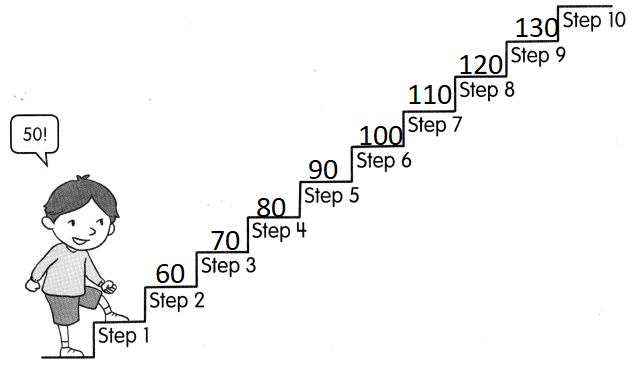# Math in Focus Grade 2 Chapter 1 Answer Key Numbers to 1,000

Go through the Math in Focus Grade 2 Workbook Answer Key Chapter 1 Numbers to 1,000 to finish your assignments.

## Math in Focus Grade 2 Chapter 1 Answer Key Numbers to 1,000

Math Journal

Count on or count back.

Question 1.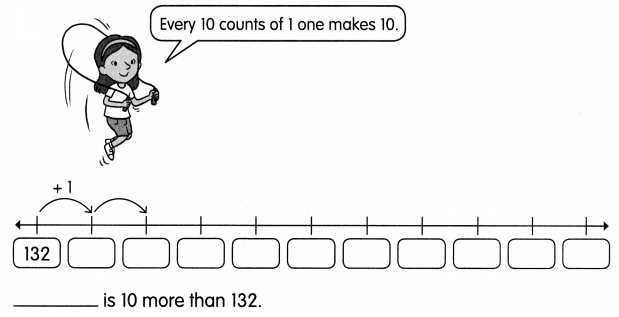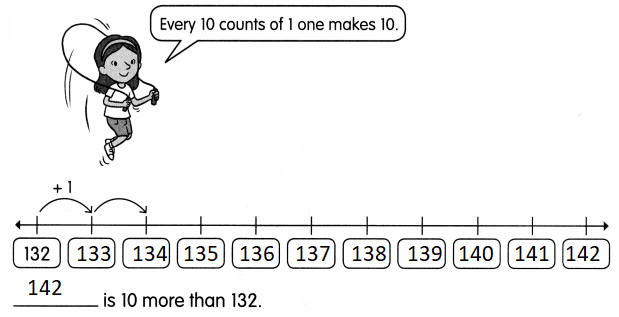Explanation:
For every number 1 is added to get 10 more of 132 that is 142

Question 2.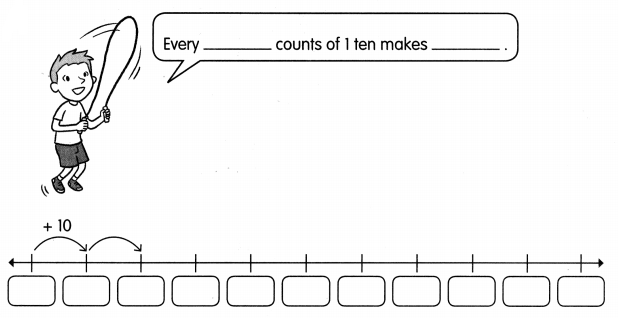___ is 100 more than ____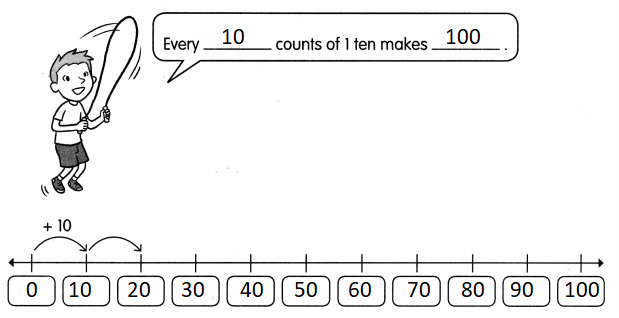Explanation:
For every number 10 is added to get 100 more of 0 that is 100

challenging Practice

Sunny Snake has swallowed some eggs. The eggs have numbers that follow a pattern. Find the missing numbers.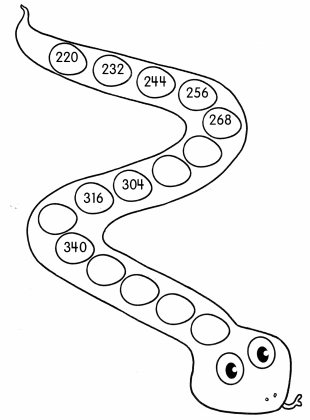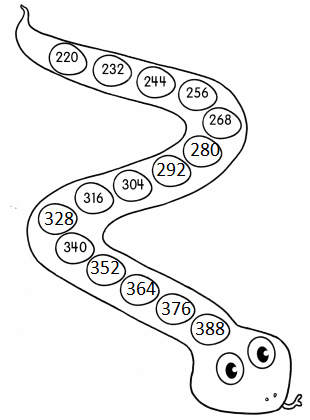Explanation:
The pattern described in snake is add + 12
For every number 12 is added

Problem Solving

Sally and Hans started counting at the same time. Sally counted on by tens from 300. Hans counted back by hundreds. After six counts, they had reached the same number What number did Hans start counting from?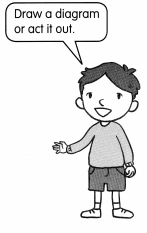Sally’s counting:
300 + 10 = 310
310 + 10 = 320
320 + 10 = 330
330 + 10 = 340
340 + 10 = 350
350 + 10 = 360
Hans counting
960 he started counting
960 – 100 = 860
860 – 100 = 760
760 – 100 = 660
660 – 100 = 560
560 – 100 = 460
460 – 100 = 360
So, they reached at 360

Chapter Review/Test

Vocabulary

Question 1.
Match.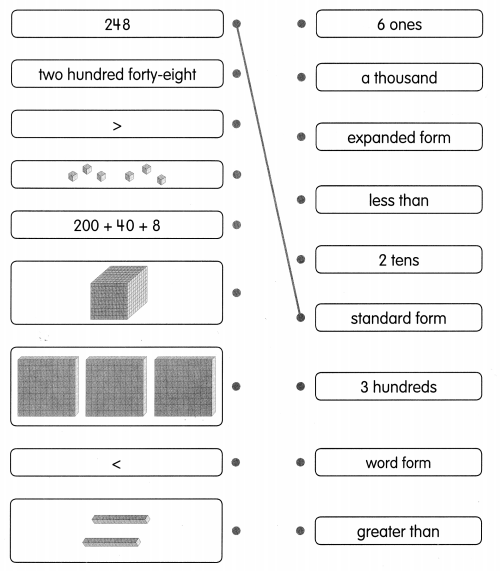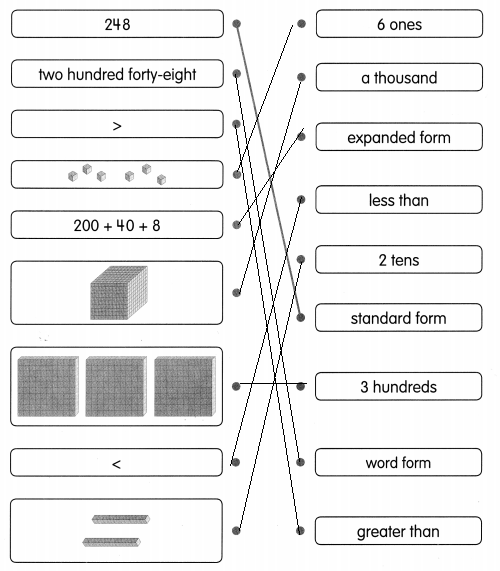Explanation:
Vocabulary is matched with the appropriate images.

Concepts and Skills

Fill in the blank.

Question 2.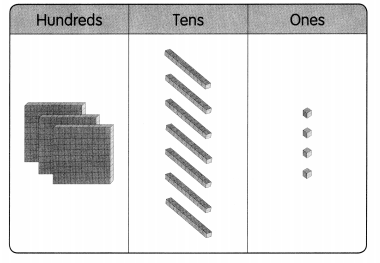The number shown above is ____
The number shown above is 376
Explanation:
1 cube = 100; 3 x 100 = 300
1 line = 10; 7 x 10 = 70
1 dots = 1; 4 x 1 = 4
300 + 70 + 4 = 374

Fill in the blanks.

Question 3.
Five hundred forty-nine is the ___ form of 549.
Five hundred forty-nine is the word form of 549.

Question 4.
The standard form of 549 is ___ and the expanded form is ___.
The standard form of 549 is 549 and the expanded form is  500 + 40 + 9

Question 5.
In 549, the digit 4 is in the __ place, the digit ___ is in the hundreds place, and the digit 9 is in the ___ place.
In 549, the digit 4 is in the tens place, the digit 5 is in the hundreds place, and the digit 9 is in the ones place.

Find the missing numbers or words.

Question 6.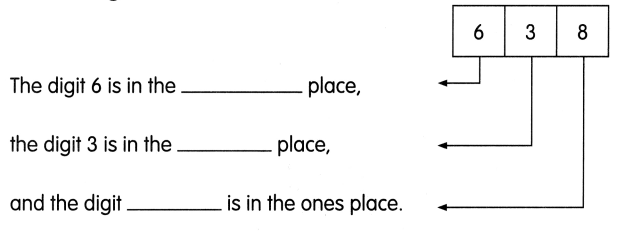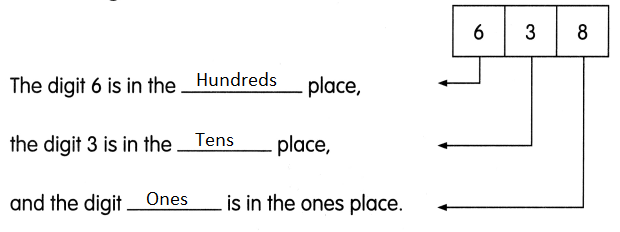Explanation:
Place value is the value of each digit in a number
Place value can be defined as the value represented by a digit in a number on the basis of its position

Fill in the blanks. Use the items in the box to help you.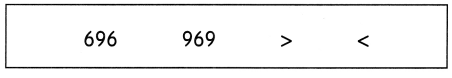Question 7.
_______ is greater than ____.
969 is greater than 696.
Explanation:
The words are used from vocabulary table

Question 8.
696 ___ 969
696 < 969
Explanation:
The symbols are used from vocabulary table

Question 9.
969 ____ 696
969 > 696
Explanation:
The symbols are used from vocabulary table

Write the numbers in order from least to greatest.

Question 10.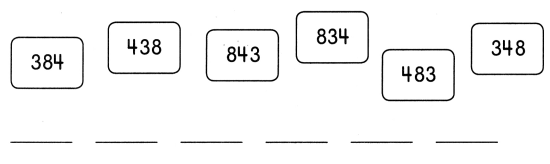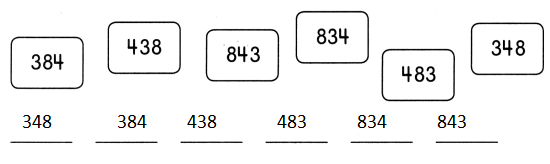Explanation:
Always start with the largest number. Always make sure the numbers are in order from largest to smallest. Always end with the smallest number

Find the missing numbers.

Question 11.
977 ____ 957 947 ___ ___ 917
977 967 957 947 937 927 917
Explanation:
In the above pattern tens place is decreased by 1
that means ten

Problem Solving

Solve.

Question 12.
Taisha has 654 stickers. Jan has 564 stickers and Pedro has 645 stickers. Who has the most stickers and who has the least stickers?
___ has the most stickers and ___ has the least stickers.
Taisha has the most stickers and Jan has the least stickers.
Explanation:
Taisha has 654 stickers.
Jan has 564 stickers and Pedro has 645 stickers.
Taisha has more and Pedro has 645
and they are having more than Jan

Question 13.
Winston climbed some steps. With each step he took, he counted on by tens. He started counting from the number 50 (Step 1) and stopped when he counted to 120.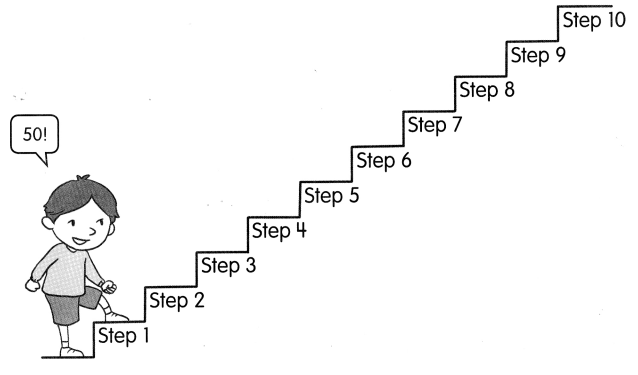He stopped at step ____